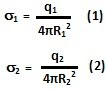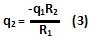## Saturday, November 27, 2010

### Irodov Problem 3.65Since electrons are free to move about within a conductor, and two electrons repel from each other, they will tend to spread themselves uniformly across the surface of the conductor. Given the spherical symmetry of the problem, the spherical shells will have a uniform charge density. Thus, the surface charge densities of the spheres will beThe electric potential at the center of the sphere O, will be due to each of the two spheres, equal to,If this potential Vo is zero then from (2) we have,Consider a spherical Gaussian surface within the innermost sphere (G1 as shown in the figure). Since there is no charge within this sphere, there must be no electric flux through G1, in other words the net electric filed inside the sphere will be zero at all points.

Consider another spherical Gaussian surface (G2 as shown in the figure) with radius r such that. Since the net charge inside this sphere is q1, the electric field in this region can be determined using Gauss law and is given by,Now consider another spherical Gaussian surface (G3 as shown in the figure) with radius r such that. The net charge within this Gaussian surface is. Hence, from Gauss law, the electric field in this region is given by,The electric potential V(r) as a function of distance is given by,
Using (4a) and (4b) we have,

#### 1 comment:

1.In the inner most gaussian sphere charge is zero means net flux comming out of the sphere is zero and not that electric field is zero.
Please explain how electric field inside a charged sphere is zero